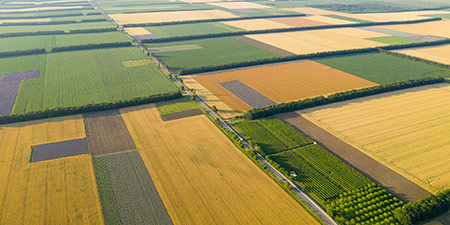# Acre (ac) to Hectar (ha)

Area Units ﹣ Converter Acre to Hectar

Here you can convert the Area unit Acre into the unit Hectar and vice versa you can convert Hectar into Acre. By clicking the "Swap units" icon, you will always obtain the desired conversion in the calculation result, i.e., ac to ha or ha to ac. With the following calculator you can also calculate any other Area unit.

An acre (ac) is equal to one 160th part of a quarter section or one 640th part of a square mile. Thus, the acre has the area of a square with a side length of about 63.62 meters.

1 ac ≈ 63,62 m × 63,62 m

The unit acre (Old English æcer acre, field) originally referred to a strip of land that could be plowed in one day with a team of oxen.

The unit of area known as the acre is now a common unit for subdividing land areas, especially in America. These are initially divided into square miles (sections) and into quarters of these sections, namely quarters. A quarter section, in turn, consists of 160 acres. In the Anglo-American world, the size of properties in land and property surveys is indicated solely by the two area units acre and square foot.

1/640 square mile = 1/160 quarter = 1 acre = 4.840 square yards = 43.560 square feet = 6.272.640 square inches = 4.046,85642 m²

One hectare (ha) is equal to the area of a square with sides 100 meters long.

1 ha = 100 m × 100 m

The hectare belongs to the International System of Units (SI), because it is a unit of measurement of area derived from a multiple of the meter or square meter.

The hectare is most commonly used in agriculture and forestry, where units of 100 by 100 meters, or 10,000 square meters, are in practical use.

Typical orders of magnitude measured or represented in hectares are, for example, the area of a polo field with 4.86 ha or with 1.5 ha the footprint of St. Peterss Basilica. It is also often asked: how many soccer fields are one hectare? The size of a standard soccer field is 0.714 hectares.

## Basis for conversion Acre (ac) to Hectar (ha) and vice versa

The abbreviation for the "Area unit Acre" is ac. The abbreviation for the "Area unit Hectar" is ha.

## Formula for the conversion of Acre (ac) to Hectar (ha) and vice versa

The calculation from Acre to Hectar shall be made using the following conversion formula:

Conversion formula Acre to Hectar

Determine the number of Hectar from Acre

Acre × 0.40468564224

## Formula for the conversion of Hectar (ha) to Acre (ac)

The calculation from Hectar to Acre shall be made using the following conversion formula:

Conversion formula Hectar to Acre

Determine the number of Acre from Hectar

Hectar × 2.4710538146717

## Overview table : How many Acre are in a Hectar ?

Acre ac ⇒ Hectar ha
0.01 ac  are  0.00404 ha
0.02 ac  are  0.00809 ha
0.03 ac  are  0.01214 ha
0.04 ac  are  0.01618 ha
0.05 ac  are  0.02023 ha
0.06 ac  are  0.02428 ha
0.07 ac  are  0.02832 ha
0.08 ac  are  0.03237 ha
0.09 ac  are  0.03642 ha
0.10 ac  are  0.04046 ha
0.20 ac  are  0.08093 ha
0.30 ac  are  0.12140 ha
0.40 ac  are  0.16187 ha
0.50 ac  are  0.20234 ha
0.60 ac  are  0.24281 ha
0.70 ac  are  0.28328 ha
0.80 ac  are  0.32374 ha
0.90 ac  are  0.36421 ha
1 ac  corresponds to  0.40468 ha
2 ac  are  0.80937 ha
3 ac  are  1.21405 ha
4 ac  are  1.61874 ha
5 ac  are  2.02342 ha
6 ac  are  2.42811 ha
7 ac  are  2.83279 ha
8 ac  are  3.23748 ha
9 ac  are  3.64217 ha
10 ac  are  4.04685 ha
20 ac  are  8.09371 ha
30 ac  are  12.14056 ha
40 ac  are  16.18742 ha
50 ac  are  20.23428 ha
60 ac  are  24.28113 ha
70 ac  are  28.32799 ha
80 ac  are  32.37485 ha
90 ac  are  36.42170 ha
100 ac  are  40.46856 ha
200 ac  are  80.93712 ha
300 ac  are  121.40569 ha
400 ac  are  161.87425 ha
500 ac  are  202.34282 ha
600 ac  are  242.81138 ha
700 ac  are  283.27995 ha
800 ac  are  323.74851 ha
900 ac  are  364.21707 ha
1 000 ac  are  404.68564 ha

## Overview table : How many Hectar are in a Acre ?

Hectar ha ⇒ Acre ac
0.01 ha  are  0.02471 ac
0.02 ha  are  0.04942 ac
0.03 ha  are  0.07413 ac
0.04 ha  are  0.09884 ac
0.05 ha  are  0.12355 ac
0.06 ha  are  0.14826 ac
0.07 ha  are  0.17297 ac
0.08 ha  are  0.19768 ac
0.09 ha  are  0.22239 ac
0.10 ha  are  0.24710 ac
0.20 ha  are  0.49421 ac
0.30 ha  are  0.74131 ac
0.40 ha  are  0.98842 ac
0.50 ha  are  1.23552 ac
0.60 ha  are  1.48263 ac
0.70 ha  are  1.72973 ac
0.80 ha  are  1.97684 ac
0.90 ha  are  2.22394 ac
1 ha  corresponds to  2.47105 ac
2 ha  are  4.94210 ac
3 ha  are  7.41316 ac
4 ha  are  9.88421 ac
5 ha  are  12.35526 ac
6 ha  are  14.82632 ac
7 ha  are  17.29737 ac
8 ha  are  19.76843 ac
9 ha  are  22.23948 ac
10 ha  are  24.71053 ac
20 ha  are  49.42107 ac
30 ha  are  74.13161 ac
40 ha  are  98.84215 ac
50 ha  are  123.55269 ac
60 ha  are  148.26322 ac
70 ha  are  172.97376 ac
80 ha  are  197.68430 ac
90 ha  are  222.39484 ac
100 ha  are  247.10538 ac
200 ha  are  494.21076 ac
300 ha  are  741.31614 ac
400 ha  are  988.42152 ac
500 ha  are  1 235.52690 ac
600 ha  are  1 482.63228 ac
700 ha  are  1 729.73767 ac
800 ha  are  1 976.84305 ac
900 ha  are  2 223.94843 ac
1 000 ha  are  2 471.05381 ac

## Source information

As source for the information in the 'Area Units' category, we have used in particular:

## Last update

This page of the 'Area Units' category was last edited or reviewed by Michael Mühl on February 20, 2023. It corresponds to the current status.

### Changes in this category "Area Units"

• Publication of Area Converter together with associated texts.